American Journal of Applied Scientific Research
Volume 2, Issue 5, September 2016, Pages: 29-32

A New Pseudorandom Sequence

Sun Quanling, Lv Hong, Chen Wanli, Qi Peng

Department of Electronic and Information Engineering, Anhui Jianzhu University, HeFei, China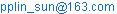(Sun Quanling)

Sun Quanling, Lv Hong, Chen Wanli, Qi Peng. A New Pseudorandom Sequence. American Journal of Applied Scientific Research. Vol. 2, No. 5, 2016, pp. 29-32. doi: 10.11648/j.ajasr.20160205.12

Received: August 30, 2016; Accepted: October 5, 2016; Published: November 21, 2016

Abstract: The m-sequence is a type of pseudorandom sequence that has been studied intensively. However, its security is threatened due to its low linear complexity. Based on the state transition rules of the m-sequence, our research group has proposed a new sequence by changing its state transition order. This provides a large number of sequences with similar levels of pseudo-randomness and autocorrelation as the m-sequence. Additionally, its linear complexity is greatly improved.

Keywords: m-sequence, State Transition, Conjugate State Pair, m-subsequence, Linear Complexity

Contents

1. Introduction

Claude Elwood Shannon, the father of information theory, proved in his two theses that the "one-time pad" cryptosystem is theoretically completely safe . However, there are limitations to this cryptosystem in practical applications. In contrast, a pseudorandom sequence-based stream cipher has additional advantages, such as easy realization, flexible length change and limited error transfer. Therefore, the pseudorandom sequence-based stream cipher has become the universal cryptosystem today. The m-sequence, which is generated by a linear feedback shift register, has the longest period with good autocorrelation, balance and running properties, and is widely used in security fields, such as spread spectrum communication and encryption. However, due to the wide application of the B-M algorithm, the structure of the linear shift register can be easily revealed through calculation. Moreover, due to its low linear complexity, the m-sequence is an easy target for attack. In applications, the m-sequence generally requires transformation to add nonlinearity, which requires additional hardware implementation complexity.

2. Application of Pseudorandom Sequence in Communication Security

In mobile communications, the encryption algorithm used for uplink communication, when the GSM system is connecting from the mobile terminal to the base station, is the A5 sequence cryptographic algorithm, which was developed by French in 1989. The A5 algorithm consists of three linear feedback shift registers (LFSR), which each use independent clock control. The A5 algorithm is suitable for high-efficiency hardware implementations, and passes known statistical tests. Although it has been reported in 2000 and 2003 that the encryption key can be cracked under part known-plaintext, this crack requires a large number of advance calculations. Therefore, it is safe for general applications.

At present, there are A5/1, A5/2 and A5/3 algorithms. The disadvantage of the A5 sequence cryptographic algorithm is its short register, so it cannot withstand a brute-force attack. However, it has been proven to be safe if a longer register or a dense feedback polynomial cryptographic algorithm is adopted.

3. Research Work

Mr. Gao Hongxun gave a Method and Proof of n Level M Sequence and its Feedback Function by subsequent state of state transition's . Mr. Taejoo Chang calculated the number of conjugate states in pairs, but there was not further research work of him. There was not study on the new sequences formed by changing the state transition. Pro. Lv Hong gave the sequence by changing the number of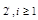pair of cross-conjugate states named m sub-sequence. There described the properties of this sequence in [3,4,8,9,14]. In this paper, the characteristics of cryptography of the m sub-sequence like randomness and linear complexity are very good. It has strong ability to resist linear attacks.

3.1. State Transition of m- sequence

The state transition rule of the largest linear feedback shift register at level n is determined by its feedback function. The first order of the m-sequence is generated when the state transforms from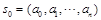; the next order of the m-sequence is generated when s1 transforms to; and the transitions are then repeated as follows: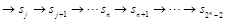(as shown by the dotted line in Fig. 1) until a full circuit is completed and a circle is formed with the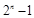period. At this stage, the transformation will output an m-sequence with a period of. This circle is also called a state transition diagram, or a state diagram for short. In this state diagram, s0 is called the original state, and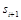is called the subsequent state of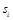.Fig. 1. State transitions of m-sequence and new sequence.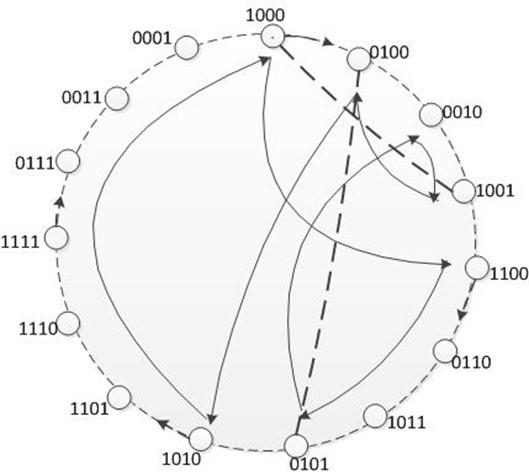Fig. 2. New state transition of.

The dotted line in Fig. 1 represents the state transition of generating an m-sequence. In these state diagrams, there are state pairs with low orders, reciprocal orders and the same orders as other orders, such as, 0101 and 0100, 0100 and 0101, etc. As shown in Fig. 1, these state pairs are called conjugate states, represented byand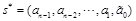. If the line between two pairs of conjugate states crosses in the transition diagram, the subsequent state of the two pairs of conjugate states can be changed to form a new state transition with the same period. At the same time for the state transition, the first order is generated and a new sequence with period of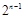is formed. As shown in Fig. 1, a full line denotes a state transition to a new state, and the state transition period will not change. For example, the primitive polynomial is, and the output sequence of the linear shift register with an original state of 1000 is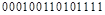. As shown in Fig. 2, 1000 and 1001, 0100 and 0101 are two pairs of cross-conjugate states, as represented by the dotted line in Fig. 2, where (1000, 1001) and (0100, 0101) are a pair of cross-conjugate states. Every subsequent state to this conjugate state pair is called the subsequent state of its conjugate state. A new state transition diagram is then formed, as shown by the solid line in Fig. 2, and its output sequence is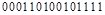.

The m-sequence has many conjugate states in the state transition diagram. It can be observed from the diagram that changing the subsequent state of the cross-conjugate states will form a new sequence with the same period. In the new sequence, the numbers of 0 and 1 are not changed. Different runs and their numbers are also not changed. By calculating the number and distribution of each run for a large number of new sequences, it can be found that the new sequence has the same balance and statistical properties as the m-sequence.

3.2. m-subsequence

The new sequence, which is called m-subsequence , is obtained by state transition of the m-sequence with the same feedback function as before. By changing the feedback polynomial of the m-sequence’s shift register, the state transition of the new sequence can be obtained. The feedback polynomial of the m-subsequence can be denoted by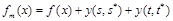, whereis the feedback polynomial and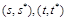is the cross-conjugation state pair which complements the conjugation state pairof the m-sequence feedback polynomial, i.e.,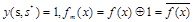and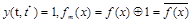. The other state’s feedback remains the same, i.e.,. The difference between the m-subsequence shift register and the m-sequence shift register is that function y has been added to the feedback function of the m-subsequence shift register. The logical relationship of function y is a nor or exclusive relationship, and the factors of the nor relationship increase with the number of registers. Nor-logic is the easiest logical circuit to realize, and a programmable device using y logic can realize the shift register of the m-subsequence. Figure 4 shows the circuit of the m-subsequence. This has been the result of intensive study by our research group, and an invention patent with patent number: CN102736892A has been obtained. The m-subsequence can also be realized using software methods, and study  describes an algorithm for the conjugate state pair. and finds the conjugate state pair and m-subsequence using C programming language.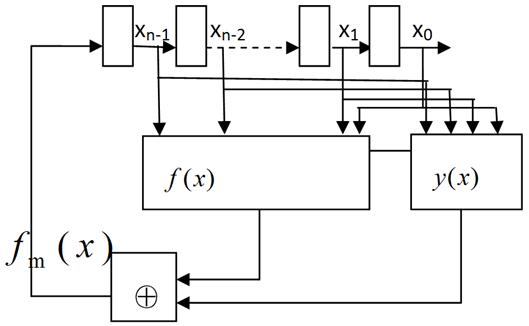Figure 3. Circuit diagram generated from m-subsequence.

3.2.1. The Number of m-subsequences

One n-level linear feedback shift register (LFSR) can only generatetranslation non-equivalent m-sequences. During the state transition process for each m-sequence, the cross-conjugate state pair and change in the order of the state transition can be found, and thus multiple different sequences can be obtained. The number of cross-conjugate state pairs increases largely with the levels of shift registers, thus more new sequences can be generated. For an m-sequence with period, there are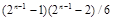cross-conjugate state pairs  in its shift register’s state transition diagram.

The following table shows the number of cross-conjugate state pairs for a n-level m sequence’s state transition diagram. The number of cross pairs represents the number of state transition changes, which can be used to form the same number of sequences with the same period. This indicates that a small increase in hardware can obtain many new sequences in an n-level linear feedback shift register (LFSR).

Table 1. Cross-conjugate state pairs in an n-level LFSR.

 Level n m sequence number Conjugate cross pairs Level n m sequence number Conjugate cross pairs Level n m sequence number Conjugate cross pairs 1 1 0 6 6 155 11 176 174251 2 1 0 7 18 651 12 144 608027 3 2 1 8 16 2667 13 630 1794155 4 2 7 9 48 10795 14 756 11180715 5 6 35 10 60 43435 15 1800 44731051

3.2.2. The Linear Complexity of the m-subsequence

The linear complexity of an n-level m sequence is relatively low. A 2n-bit cipher text can be obtained by an analyzer through solving a linear system of equations or adopting the B-M algorithm to find the characteristic polynomial of the m-sequence, which will also provide the structure of the shift register. Hence, although an m-sequence has the longest sequence period and good random statistical properties, it cannot be regarded as a key-stream sequence.

The m-subsequence is a nonlinear sequence that changes the output order of the m-sequence and thus changes its linearity, and therefore can enhance its security and make cryptanalysis more difficult. The B-M algorithm is adopted to calculate the complexity of new sequences. Table 2 shows that the linear complexity of an m-subsequence with the same level is higher than that of the m-sequence, with an exponential growth relationship.

Table 2. Complexities of the m-sequence and m-subsequence with the same level.

 Level n 5 7 8 9 10 11 15 17 Linear complexity of m sequence 5 7 8 9 10 11 15 17 Linear complexity of m subsequence 17 64 128 298 513 1028 9165 65532

Linear complexity is a very important property for a key-stream’s pseudorandom sequence. The higher the complexity is, the stronger the anti-attack ability will be. Under conditions where new hardware is added, after the m-subsequence reconstructs the m-sequence, it will then generate a new nonlinear sequence. Thus, the linear complexity has been greatly improved, and the statistical properties have also been maintained, and thus this method can be applied to key streams.

3.2.3. Autocorrelation of m-subsequence

With high randomness, the m-sequence has similar autocorrelation to white noise, and is applicable as a key stream. After making state changes for many m-sequences with the same level, the autocorrelation coefficient can be calculated for different m-subsequences with the same period. The m-subsequence has similar autocorrelation to the m-sequence. Fig. 4 shows that the same algorithm is used to calculate the autocorrelation coefficient values of the m-sequence and its corresponding m-subsequence. The same level m-sequence can be used to form many subsequences, and its autocorrelation is not as obvious as the bivalence of the m-sequence, but it is close to bivalent.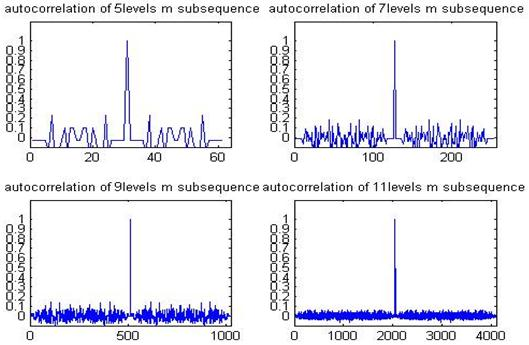Fig. 4. some level m-subsequence autocorrelation.

4. Application of m-subsequence in Cryptography

During the signal encryption process of mobile communication, a simple but efficient method is required to enable real time encryption rapidly, requiring hardware of low complexity that can be realized easily. The A5 algorithm applied in traditional GSM encryption applications which works perfectly. The m-subsequence is a simple improvement at the same level as the m-sequence, which does not change the randomness but greatly improves complexity and provides more available sequences. The research team is also working on other properties of m-subsequence, and it is believed that the m-sub sequence can be widely used in the communication field.

References

1. Behrouz A. Forouzan. Cryptography and Network Security [M]. BeiJing, Tsinghua. 2009: 242-244.
2. Gao Hongxun,A Method and Proof of n Level M Sequence and its Feedback Function [J]. ACTA MATHEMATICAE APPLACATAE SINICA. 2(4), 1979, 11, 316-324. (in chinese).
3. LV Hong, DUAN Ying-ni, GUAN Bi-cong, et al. Construction of First Class of m subsequences [J]. Acta Electronica Sinica, 35(10), 2007.10, 2029 -2031. (in chinese).
4. LV Hong, ZHANG Ai-xue, et al. Study of the Non-linear Feedback Functions and a class Subsequence Base on the Root-functions [J]. Acta Electronica Sinica, 40(10), 2012, 10, 2127-2132. (in chinese).
5. Taejoo Chang, Iickho Song, Sung Ho Cho. Some Properties of Cross Join Pairs in Maximum Length Linear Sequences. [c] 1990 ternational Symposium on Information Theory and Its Applications, Hawaii, U.S.A., November 27-30
6. Goresky M, Klapper A, Algebraic Shift Register Sequences [M], Cambridge University Press, 2012.
7. Tor Helleseth, Torleiv Klove, The number of Cross-Join Pairs in Maximum Length Linear Sequences. IEEE TRANSACTIONS ON INFORMATION THEORY, VOL. 3, NO. 6, NOVEMBER 1991.1731-1733.
8. Lv Hong, Xie Jianxia, Qi Peng. Generating of A Nonlinear Pseudorandom sequence Using Linear Feedback Shift Register [C]. Proceedings of 2012 International Conference on ICICT, 2012, 10, 432-435.
9. LV Hong, QI Peng et al. Constuction and Analysis of a Class Non-linear Spread Spetrum Sequence [J]. Acta Electronica Sinica, 41(10), 2013.10, 1939-1943. (in chinese).
10. FANG Jun-chu, LV Hong et al. C language implementation of a nonlinear sequence generated by m sequence [J].Journal of Henan University of Science and Technology: Natural Science Edition. 34(6), 2013, 12, 47-49. (in chinese).
11. GAO Jun-tao, Study on Design and Analysis of Pseudorandom Sequences [D]. Xian, XIDIAN UNIVISITY, 2006. (in chinese)
12. LUO Qi-bin, ZHANG Jian, Status Quo and Development of Stream Cipher [J]. Information and Electronic Engineering, 4(1), 2006, 2, 75-79. (in chinese).
13. FENG Deng-guo, Status quo and trend of cryptography [J]. Journal of China Institute of Communications, 23(5) 2002, 5, 18-26.
14. LV Hong. Design and Implement of A Maximal Length Nonlinear Pseudorandom Sequencs [A]. Proceedings of the 2009 International Conference on Computer and Communications Security [C]. Hong Kong. China. 2009: 64-67.

 Contents 1. 2. 3. 3.1. 3.2. 4.
Article ToolsAbstractPDF(1255K)